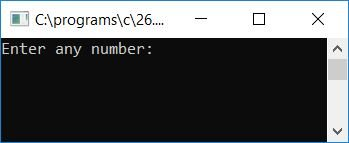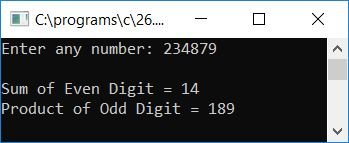# C Program to Print the Sum of Even and the Product of Odd Digits

In this tutorial, we will learn about how to create a program in C that will ask the user to enter any number as input and then find and print the sum of the even digits and the product of the odd digits present in that number.

The question is: write a program in C to print the sum of even digits and product of odd digits of any given number given by the user at run-time. Here is the program:

```#include<stdio.h>
#include<conio.h>
int main()
{
int num, evenSum=0, oddProd=1, rem, temp;
printf("Enter any number: ");
scanf("%d", &num);
while(num>0)
{
rem = num%10;
if(rem%2==0)
evenSum = evenSum + rem;
else
oddProd = oddProd * rem;
num = num/10;
}
printf("\nSum of Even Digit = %d", evenSum);
printf("\nProduct of Odd Digit = %d", oddProd);
getch();
return 0;
}```

As the above program was written in the Code::Blocks IDE, here is the sample run after a successful build and run:Supply any number, say 234879, and press the ENTER key to see the sum of even digits (that is, 2, 4, and 8) and the product of odd digits (that is, 3, 7, and 9). As the digits 2, 4, and 8 are the three even numbers, and 3, 7, and 9, the three odd numbers. As a result, the program will compute the sum of the even and odd digits (numbers). Here is the second snapshot of the sample run:Here are some of the main steps used in the above program:

• Receive any number from the user at runtime, say 234879.
• Now create a while loop that runs until the number (say 234879) is greater than 0.
• Within the while loop, assign num%10, 234879%10, or 9 to the variable "rem." Here we have calculated the digit (last digit) of the given number by using the modulus or remainder operator (%).
• Now we have to check that the remainder is an even number or an odd number.
• If it is an even number, then sum it up.
• Here we have used the variable evenSum to store the summation result of the even digit of the given number.
• Never forget to set the variable evenSum to 0 at the start of the program.
• If the number is found to be an even number (using the if block), just initialize evenSum+rem to the evenSum variable.
• And if the number is found to be an odd number, then just initialize oddProd*rem to the variable oddProd. Never forget to initialize 1 to the variable, say oddProd, at the start of the program.
• For example, if the user has entered 234879 as an input. Then, at the first run of the while loop, num%10, 234879%10, or 9 will be initialized to rem. Because 9 is an odd number, oddProd*rem, 1*9, or 9 will be initialized to oddProd.
• After doing the above steps, divide the number with 10, that is, initialize num/10 or 234879/10 or 23487 to num.
• At the second run of the while loop, num%10 or 23487%10 or 7 will be initialized to rem, as 7 is also an odd number, then oddProd*rem or 9*7 or 63 will be initialized to oddProd, and again divide the number with 10 and continue for the next check.
• Do the same operation until the number becomes 0.
• In this way, after coming out of the while loop, we have two variables, say evenSum and oddProd, that hold up the sum of the even digits and the product of the odd digits of the given number, say 234879.
• Finally, print the value of both variables.

C Quiz

« Previous Program Next Program »